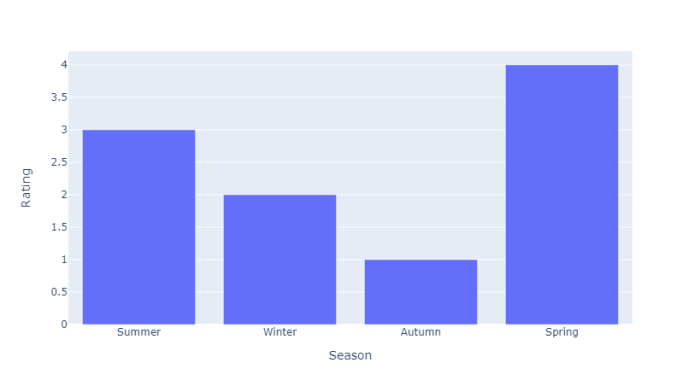# How to change the size of a Dash Graph in Python Plotly?

Plotly supports two different libraries "Plotly graphs in a Dash app" and "Plotly Graph objects in Plotly Express". Dash is a Python framework and it is used to create interactive web-based dashboard applications. The dash library adds all the required libraries to web-based dashboard applications

Import dash core components and html components. Add plotly.express method to generate graphs. Use the Dcc.Graph() method to set the style for height and width coordinates.

In this tutorial, we will show how you can add multiple graphs to a Plotly Dash app on a single browser page. Follow the steps given below to generate Dash app on a single page.

Step 1

Import the Dash library.

import dash

Step 2

Import the Dash core components, dcc and html

from dash import dcc, html

Step 3

Import the dash dependencies using the below module −

from dash.dependencies import Input, Output

Step 4

Import the plotly.express module and alias as px

import plotly.express as px

Step 5

Generate dataset using the Pandas module. Let us generate the dataset using the following method,

# import pandas module
import pandas as pd

df_bar = pd.DataFrame({
"Season": ["Summer", "Winter", "Autumn", "Spring"],
"Rating": [3,2,1,4]
})

Step 6

Generate the bar chart using the following coordinates and store it inside the fig variable.

fig = px.bar(df_bar, x="Season", y="Rating", barmode="group")

Step 7

Create the main function to run the app server using the below command,

app = dash.Dash(__name__)
if __name__ == '__main__':
app.run_server(debug=True)

Step 8

Generate the app layout for html children in div sections. It is defined below,

app.layout = html.Div(children=[

# elements from the top of the page
html.Div([html.H1(children='Dash app1'),
html.Div(children='''Dash: First graph.'''),
dcc.Graph(id='graph1',figure=fig),]),

# New Div for all elements in the new 'row' of the page
html.Div([
html.H1(children='Dash app2'),
html.Div(children='''Dash: Second graph.'''),
dcc.Graph(id='graph2', figure=fig),
]),
])

## Example

Here is the complete code to change the size of a Dash Graph in Plotly −

import dash
from dash import dcc, html
import pandas as pd
import plotly.express as px

app = dash.Dash(__name__)
df_bar = pd.DataFrame({
"Season": ["Summer", "Winter", "Autumn", "Spring"],
"Rating": [3, 2, 1, 4]
})

fig = px.bar(df_bar, x="Season", y="Rating", barmode="group")

# app layout for html div and set height and width
app.layout = html.Div(children=[

# All elements from the top of the page
html.Div([
html.H1(children='Dash app1'),
html.Div(children='''
Dash: First graph.
'''),

dcc.Graph(
id='graph1',
figure=fig,
style={'width': '60vw', 'height': '70vh'}
),
])
])

if __name__ == '__main__':
app.run_server(debug=True)

## Output

It will show the following output on the console.

Dash is running on http://127.0.0.1:8050/